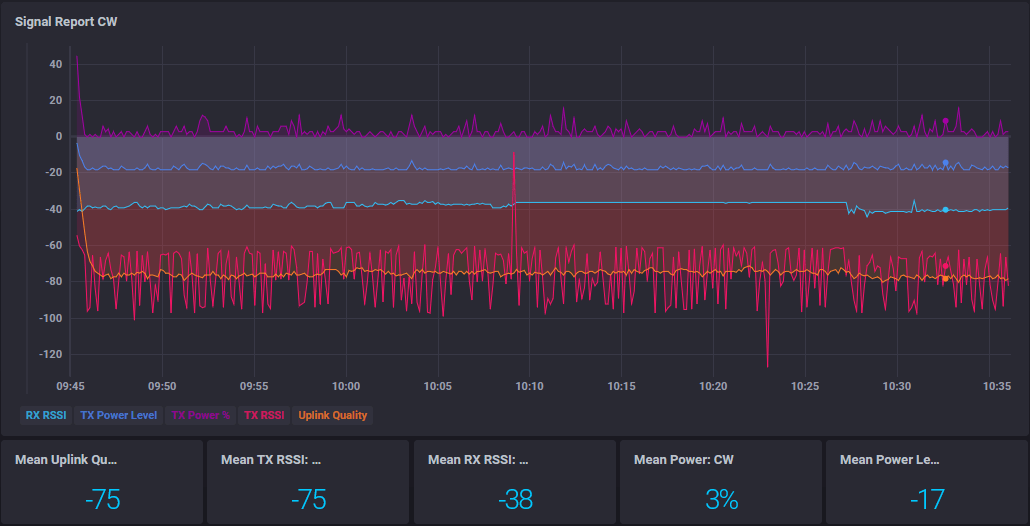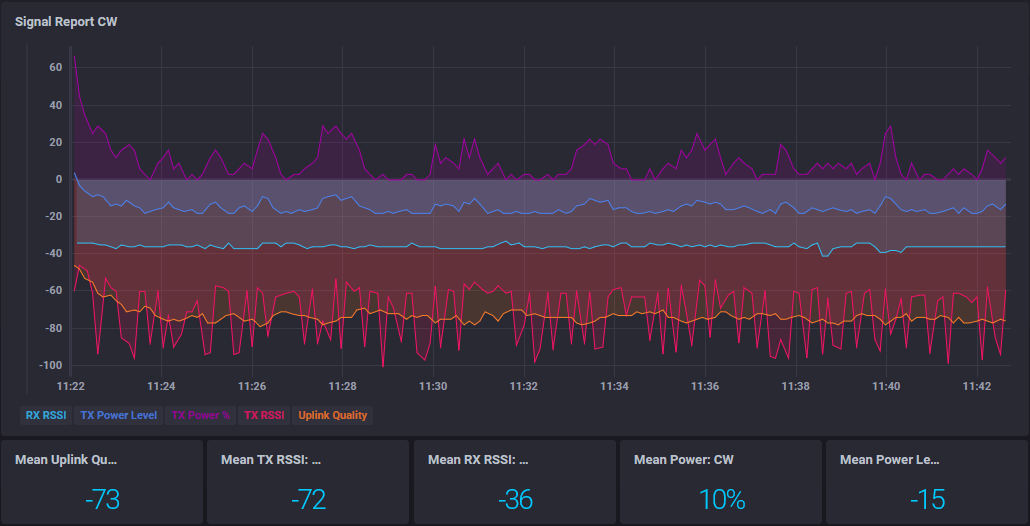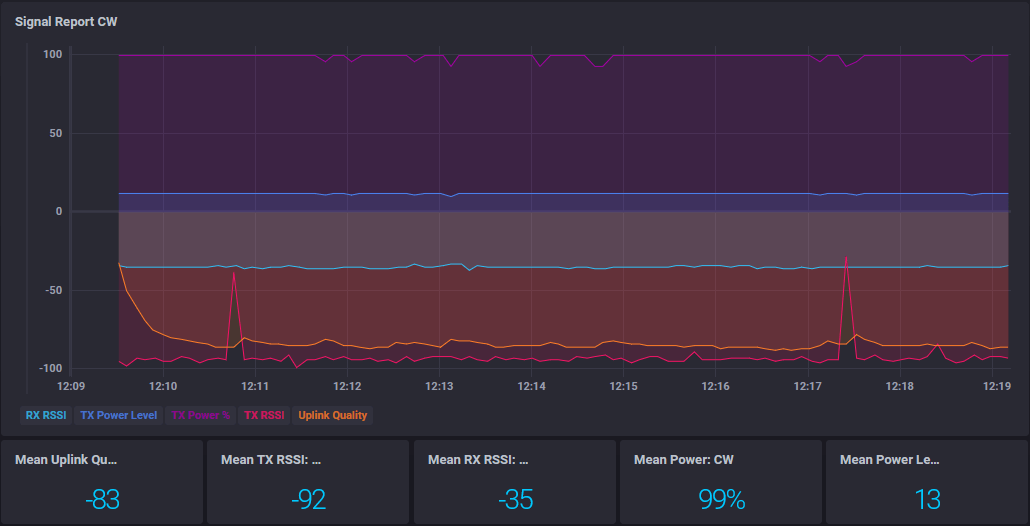# RFM69: ATC only works if MY_DEBUG_VERBOSE_RFM69 defined

• I'm currently experimenting with RFM69 radios. I've already built a well working multi-transport GW (NRF24 + RFM69) based on the current 2.4.0 dev branch - sketch and setup is posted here.

Now I'm evaluating the range / coverage of different RFM69 transceivers and antennas in and around the house, taking measurements using the RFM69_RFM95_ATC_SignalReport sketch with these defines:

``````#define MY_DEBUG
// #define MY_DEBUG_VERBOSE_RFM69

#define MY_SIGNAL_REPORT_ENABLED

// RFM69
#define MY_RFM69_NEW_DRIVER   // Required by dualRF GW
#define MY_RFM69_NETWORKID 69
``````

The multi-transport GW requires to use the new RFM69 driver, which has ATC enabled by default. As far as I understand, ATC is a technique to automatically adjust the TX power to match a specified TX signal strength (-80 dBm by default), which keeps the RF "polution" to a minimum and optimizes power consumption.

What I expect to see is a varying TX power depending on the signal quality / distance to the GW. A node right next to the GW should have a power level close to 0%. Across the house or outside, it should be much higher.

Here are some mean values collected over a period of half an hour each, using a non-amplified RFM69CW:

Same Room as GW -85 -92 -28 13 100
Floor below, two rooms across -91 -94 -71 13 100
Garden (~30m) -92 -94 -79 13 100

TX RSSI is always below the target of -80, so ATC is boosting the TX power to the highest possible level. Correct? But even when the node is close to the GW, the TX RSSI exeeds the target value. Bad antenna?

Now look what happens when I uncomment `#define MY_DEBUG_VERBOSE_RFM69` on the node:

Same Room as GW -52 -57 -29 -18 0
Floor below, two rooms across -78 -78 -63 -6 38
Garden (~30m) -72 -78 -85 11 93

These results look far more reasonable! But defining `MY_DEBUG_VERBOSE_RFM69` shouldn't make a difference, right? As soon as I remove it, I'm back to the results in the first table.

I've tried 2.3.2 and 2.4.0 on the node, they both behave the same. The RFM69 is connected to a 3V3 8 MHz Pro Mini. They are both powered with a regulated 3V3 source. `MY_RFM69_ATC_MODE_DISABLED` is not defined.

For the antennas I'm using straight solid core wires of 82mm length on both transceivers, directly soldered to the ANT pin. I don't think that this is the issue - the range and reliability of the connection is great: out of a total of 48436 messages, I got only got 21 NACKs (< 0,05%).

Am I missing something here? Is there a bug?

• Interesting finding!
I'm trying to replicate the behaviour, and have added some debug print statements in my code, which shows the tx level after each transmission. The number is changing often which doesn't correspond with your behaviour.
How did you measure this?

• @electrik I'm using the RFM69_RFM95_ATC_SignalReport sketch and store every message in a time-series database. The values listed in the table are the arithmetic means of the whole measurement period in a specific location.

I suspect that this is possibly a timing issue. And it also seems to be related to v2.4.0.

Adding a `wait(50)` right before the return statement of the `RFM69_executeATC` function in `RFM69_new.cpp` on line 636 seems to "fix" it. The TX power is now properly adjusting without defining `MY_DEBUG_VERBOSE_RFM69` and the reported TX RSSI value is no longer pinned down to a value below -90, regardless of how close the node is to the GW.

If I undefine `MY_DEBUG` aswell, I need a delay of at least 125 ms to achieve lower power levels.

Here's the results of this adjustment:TX RSSI is a little jumpy, but the connection is stable and the power level is regulated.

And just a few minutes ago, I changed my multi-transport GW running at 2.4.0 alpha to a RFM69-only GW with 2.3.2, while the RFM69 node is still on 2.4.0 alpha without my adjustments in the driver. With this change, the node is regulating the power levels aswell.

Results with the RFM69 GW at 2.3.2:EDIT: For comparison, this is the signal report of a RFM69 node with unmodified driver at 2.4.0 alpha connected to a RFM69-only GW at 2.4.0 alpha:The power level is near 100%, while the TX RSSI stays far below the target value.

• Here's a roundup of all combinations I've tried today and their results:

GW Version Node Version `MY_DEBUG_VERBOSE_RFM69` defined? `wait(50)` on node? TX power regulating?
2.4.0-alpha 2.4.0-alpha NO NO NO
2.4.0-alpha 2.4.0-alpha YES NO YES
2.4.0-alpha 2.4.0-alpha NO YES YES
2.4.0-alpha 2.4.0-alpha YES YES YES
2.4.0-alpha 2.3.2 NO NO NO
2.4.0-alpha 2.3.2 YES NO YES
2.4.0-alpha 2.3.2 NO YES YES
2.4.0-alpha 2.3.2 YES YES YES
2.3.2 2.4.0-alpha NO NO YES
2.3.2 2.3.2 NO NO YES

Only if the GW is on 2.4.0-alpha and the node (2.3.2 or 2.4.0-alpha) does neither define `MY_DEBUG_VERBOSE_RFM69` nor add `wait(50)` in `RFM69_executeATC`, the node doesn't regulate the TX power. In all other cases, it does adjust the TX power. If the GW is on 2.3.2 it always regulates.

• @BearWithBeard
thank you for your investigationsyes, this is problably a timing issue. this will be fixed asap

• Hello,
I would like to step in here as you will look into this ATC mode anywayI've been observing the behaviour, and when it successfully sends a message, the power level is decreased. Excellent.
At some point the power becomes too low and messages are not ACK'ed anymore. I had expected the power level would be increased at that point, but it doesn't.
The result is that on another succesfull delivery the power level is decreased even more, and the node can't find the gateway anymore. Then the node increases its power level to the initial value (let's say 5) and then the process starts over again.
Would it be an idea to increase the power level when there is a NACK and then try to stay around this "ideal" level?

I'm willing to test if it is needed• After I wrote the message above I realised this is another topic in fact, I hope you don't mind.
To explain a bit more what happens, here is some debug output. Each 10 seconds an increasing counter is sent to the gateway, and after each sent message the TX power is printed on the screen.

``````46219 TSM:UPL:OK
54607 TSF:MSG:SEND,31-31-0-0,s=22,c=1,t=23,pt=1,l=1,sg=0,ft=0,st=OK:4
Power is 3
64618 TSF:MSG:SEND,31-31-0-0,s=22,c=1,t=23,pt=1,l=1,sg=0,ft=0,st=OK:5
Power is 2
74629 TSF:MSG:SEND,31-31-0-0,s=22,c=1,t=23,pt=1,l=1,sg=0,ft=0,st=OK:6
Power is 1
85655 !TSF:MSG:SEND,31-31-0-0,s=22,c=1,t=23,pt=1,l=1,sg=0,ft=0,st=NACK:7
Power is 1
94650 TSF:MSG:SEND,31-31-0-0,s=22,c=1,t=23,pt=1,l=1,sg=0,ft=1,st=OK:8
Power is 0
105168 TSF:MSG:SEND,31-31-0-0,s=22,c=1,t=23,pt=1,l=1,sg=0,ft=0,st=OK:9
Power is -1
114671 TSF:MSG:SEND,31-31-0-0,s=22,c=1,t=23,pt=1,l=1,sg=0,ft=0,st=OK:10
Power is -2
124682 TSF:MSG:SEND,31-31-0-0,s=22,c=1,t=23,pt=1,l=1,sg=0,ft=0,st=OK:11
Power is -3
135708 !TSF:MSG:SEND,31-31-0-0,s=22,c=1,t=23,pt=1,l=1,sg=0,ft=0,st=NACK:12
Power is -3
144703 TSF:MSG:SEND,31-31-0-0,s=22,c=1,t=23,pt=1,l=1,sg=0,ft=1,st=OK:13
Power is -4
154714 TSF:MSG:SEND,31-31-0-0,s=22,c=1,t=23,pt=1,l=1,sg=0,ft=0,st=OK:14
Power is -5
164724 TSF:MSG:SEND,31-31-0-0,s=22,c=1,t=23,pt=1,l=1,sg=0,ft=0,st=OK:15
Power is -6
174735 TSF:MSG:SEND,31-31-0-0,s=22,c=1,t=23,pt=1,l=1,sg=0,ft=0,st=OK:16
Power is -7
184745 TSF:MSG:SEND,31-31-0-0,s=22,c=1,t=23,pt=1,l=1,sg=0,ft=0,st=OK:17
Power is -8
195772 !TSF:MSG:SEND,31-31-0-0,s=22,c=1,t=23,pt=1,l=1,sg=0,ft=0,st=NACK:18
Power is -8
204980 TSF:MSG:SEND,31-31-0-0,s=22,c=1,t=23,pt=1,l=1,sg=0,ft=1,st=OK:19
Power is -9
214990 TSF:MSG:SEND,31-31-0-0,s=22,c=1,t=23,pt=1,l=1,sg=0,ft=0,st=OK:20
Power is -10
225804 !TSF:MSG:SEND,31-31-0-0,s=22,c=1,t=23,pt=1,l=1,sg=0,ft=0,st=NACK:21
Power is -10
235438 TSF:MSG:SEND,31-31-0-0,s=22,c=1,t=23,pt=1,l=1,sg=0,ft=1,st=OK:22
Power is -11
245825 !TSF:MSG:SEND,31-31-0-0,s=22,c=1,t=23,pt=1,l=1,sg=0,ft=0,st=NACK:23
Power is -11
255836 !TSF:MSG:SEND,31-31-0-0,s=22,c=1,t=23,pt=1,l=1,sg=0,ft=1,st=NACK:24
Power is -11
265846 !TSF:MSG:SEND,31-31-0-0,s=22,c=1,t=23,pt=1,l=1,sg=0,ft=2,st=NACK:25
Power is -11
275857 !TSF:MSG:SEND,31-31-0-0,s=22,c=1,t=23,pt=1,l=1,sg=0,ft=3,st=NACK:26
Power is -11
285868 !TSF:MSG:SEND,31-31-0-0,s=22,c=1,t=23,pt=1,l=1,sg=0,ft=4,st=NACK:27
Power is -11
``````

Here are a couple of NACKs, so this is on the limit of TX power IMO. Still one succesfull one, which decreases the power even more.

``````294862 TSF:MSG:SEND,31-31-0-0,s=22,c=1,t=23,pt=1,l=1,sg=0,ft=5,st=OK:28
Power is -12
305889 !TSF:MSG:SEND,31-31-0-0,s=22,c=1,t=23,pt=1,l=1,sg=0,ft=0,st=NACK:29
Power is -12
315899 !TSF:MSG:SEND,31-31-0-0,s=22,c=1,t=23,pt=1,l=1,sg=0,ft=1,st=NACK:30
Power is -12
325910 !TSF:MSG:SEND,31-31-0-0,s=22,c=1,t=23,pt=1,l=1,sg=0,ft=2,st=NACK:31
Power is -12
335921 !TSF:MSG:SEND,31-31-0-0,s=22,c=1,t=23,pt=1,l=1,sg=0,ft=3,st=NACK:32
Power is -12
345931 !TSF:MSG:SEND,31-31-0-0,s=22,c=1,t=23,pt=1,l=1,sg=0,ft=4,st=NACK:33
Power is -12
355942 !TSF:MSG:SEND,31-31-0-0,s=22,c=1,t=23,pt=1,l=1,sg=0,ft=5,st=NACK:34
Power is -12
``````

Five failed messages and the node starts finding its parent. But also that fails.

``````356024 !TSM:READY:UPL FAIL,SNP
356073 TSM:FPAR
356089 ?TSF:MSG:SEND,31-31-255-255,s=255,c=3,t=7,pt=0,l=0,sg=0,ft=6,st=OK:
358219 TSM:FPAR
358252 ?TSF:MSG:SEND,31-31-255-255,s=255,c=3,t=7,pt=0,l=0,sg=0,ft=0,st=OK:
360382 TSM:FPAR
360415 ?TSF:MSG:SEND,31-31-255-255,s=255,c=3,t=7,pt=0,l=0,sg=0,ft=0,st=OK:
362528 TSM:FPAR
362561 ?TSF:MSG:SEND,31-31-255-255,s=255,c=3,t=7,pt=0,l=0,sg=0,ft=0,st=OK:
364675 !TSM:FPAR:FAIL
364691 TSM:FAIL:CNT=1
364724 TSM:FAIL:DIS
364740 TSF:TDI:TSL
364888 !TSF:SND:TNR
Power is -12
374784 TSM:FAIL:RE-INIT
374800 TSM:INIT
374833 TSM:INIT:TSP OK
374865 TSM:INIT:STATID=31
374898 TSF:SID:OK,ID=31
374915 TSM:FPAR
374947 ?TSF:MSG:SEND,31-31-255-255,s=255,c=3,t=7,pt=0,l=0,sg=0,ft=0,st=OK:
375046 !TSF:SND:TNR
``````

Now the Transportlayer is re-initialised and the power level goes to 5, which makes the messages being ACKed again. This starts the process all over again.

``````Power is 5
375799 TSF:MSG:FPAR OK,ID=0,D=1
377044 TSM:FPAR:OK
377061 TSM:ID
377077 TSM:ID:OK
377110 TSM:UPL
377192 TSF:MSG:SEND,31-31-0-0,s=255,c=3,t=24,pt=1,l=1,sg=0,ft=0,st=OK:1
377978 TSF:MSG:PONG RECV,HP=1
378028 TSM:UPL:OK
385105 TSF:MSG:SEND,31-31-0-0,s=22,c=1,t=23,pt=1,l=1,sg=0,ft=0,st=OK:37
Power is 3
395116 TSF:MSG:SEND,31-31-0-0,s=22,c=1,t=23,pt=1,l=1,sg=0,ft=0,st=OK:38
Power is 2
405127 TSF:MSG:SEND,31-31-0-0,s=22,c=1,t=23,pt=1,l=1,sg=0,ft=0,st=OK:39
Power is 1
415137 TSF:MSG:SEND,31-31-0-0,s=22,c=1,t=23,pt=1,l=1,sg=0,ft=0,st=OK:40
Power is 0
425148 TSF:MSG:SEND,31-31-0-0,s=22,c=1,t=23,pt=1,l=1,sg=0,ft=0,st=OK:41
Power is -1
435159 TSF:MSG:SEND,31-31-0-0,s=22,c=1,t=23,pt=1,l=1,sg=0,ft=0,st=OK:42
Power is -2
445169 TSF:MSG:SEND,31-31-0-0,s=22,c=1,t=23,pt=1,l=1,sg=0,ft=0,st=OK:43
Power is -3
455180 TSF:MSG:SEND,31-31-0-0,s=22,c=1,t=23,pt=1,l=1,sg=0,ft=0,st=OK:44
Power is -4
465207 TSF:MSG:SEND,31-31-0-0,s=22,c=1,t=23,pt=1,l=1,sg=0,ft=0,st=OK:45
Power is -5
475201 TSF:MSG:SEND,31-31-0-0,s=22,c=1,t=23,pt=1,l=1,sg=0,ft=0,st=OK:46
Power is -6
485720 TSF:MSG:SEND,31-31-0-0,s=22,c=1,t=23,pt=1,l=1,sg=0,ft=0,st=OK:47
Power is -7
495222 TSF:MSG:SEND,31-31-0-0,s=22,c=1,t=23,pt=1,l=1,sg=0,ft=0,st=OK:48
Power is -8
505446 TSF:MSG:SEND,31-31-0-0,s=22,c=1,t=23,pt=1,l=1,sg=0,ft=0,st=OK:49
Power is -9
516259 !TSF:MSG:SEND,31-31-0-0,s=22,c=1,t=23,pt=1,l=1,sg=0,ft=0,st=NACK:50
Power is -9
525254 TSF:MSG:SEND,31-31-0-0,s=22,c=1,t=23,pt=1,l=1,sg=0,ft=1,st=OK:51
Power is -10
536281 !TSF:MSG:SEND,31-31-0-0,s=22,c=1,t=23,pt=1,l=1,sg=0,ft=0,st=NACK:52
Power is -10
546291 !TSF:MSG:SEND,31-31-0-0,s=22,c=1,t=23,pt=1,l=1,sg=0,ft=1,st=NACK:53
Power is -10
556302 !TSF:MSG:SEND,31-31-0-0,s=22,c=1,t=23,pt=1,l=1,sg=0,ft=2,st=NACK:54
Power is -10
565510 TSF:MSG:SEND,31-31-0-0,s=22,c=1,t=23,pt=1,l=1,sg=0,ft=3,st=OK:55
Power is -11
576323 !TSF:MSG:SEND,31-31-0-0,s=22,c=1,t=23,pt=1,l=1,sg=0,ft=0,st=NACK:56
Power is -11
586334 !TSF:MSG:SEND,31-31-0-0,s=22,c=1,t=23,pt=1,l=1,sg=0,ft=1,st=NACK:57
Power is -11
596344 !TSF:MSG:SEND,31-31-0-0,s=22,c=1,t=23,pt=1,l=1,sg=0,ft=2,st=NACK:58
Power is -11
606355 !TSF:MSG:SEND,31-31-0-0,s=22,c=1,t=23,pt=1,l=1,sg=0,ft=3,st=NACK:59
Power is -11
615989 TSF:MSG:SEND,31-31-0-0,s=22,c=1,t=23,pt=1,l=1,sg=0,ft=4,st=OK:60
Power is -12
626376 !TSF:MSG:SEND,31-31-0-0,s=22,c=1,t=23,pt=1,l=1,sg=0,ft=0,st=NACK:61
Power is -12
636387 !TSF:MSG:SEND,31-31-0-0,s=22,c=1,t=23,pt=1,l=1,sg=0,ft=1,st=NACK:62
Power is -12
646397 !TSF:MSG:SEND,31-31-0-0,s=22,c=1,t=23,pt=1,l=1,sg=0,ft=2,st=NACK:63
Power is -12
656408 !TSF:MSG:SEND,31-31-0-0,s=22,c=1,t=23,pt=1,l=1,sg=0,ft=3,st=NACK:64
Power is -12
666419 !TSF:MSG:SEND,31-31-0-0,s=22,c=1,t=23,pt=1,l=1,sg=0,ft=4,st=NACK:65
Power is -12
676429 !TSF:MSG:SEND,31-31-0-0,s=22,c=1,t=23,pt=1,l=1,sg=0,ft=5,st=NACK:66
Power is -12
676560 TSM:FPAR
676577 ?TSF:MSG:SEND,31-31-255-255,s=255,c=3,t=7,pt=0,l=0,sg=0,ft=6,st=OK:
678707 TSM:FPAR
678739 ?TSF:MSG:SEND,31-31-255-255,s=255,c=3,t=7,pt=0,l=0,sg=0,ft=0,st=OK:
680869 TSM:FPAR
680902 ?TSF:MSG:SEND,31-31-255-255,s=255,c=3,t=7,pt=0,l=0,sg=0,ft=0,st=OK:
683016 TSM:FPAR
683048 ?TSF:MSG:SEND,31-31-255-255,s=255,c=3,t=7,pt=0,l=0,sg=0,ft=0,st=OK:
685162 !TSM:FPAR:FAIL
685178 TSM:FAIL:CNT=1
685211 TSM:FAIL:DIS
685228 TSF:TDI:TSL
685375 !TSF:SND:TNR
Power is -12
695271 TSM:FAIL:RE-INIT
695287 TSM:INIT
695320 TSM:INIT:TSP OK
695353 TSM:INIT:STATID=31
695386 TSF:SID:OK,ID=31
695402 TSM:FPAR
695435 ?TSF:MSG:SEND,31-31-255-255,s=255,c=3,t=7,pt=0,l=0,sg=0,ft=0,st=OK:
695533 !TSF:SND:TNR
Power is 5
696434 TSF:MSG:FPAR OK,ID=0,D=1
697548 TSM:FPAR:OK
697565 TSM:ID
697581 TSM:ID:OK
697614 TSM:UPL
697696 TSF:MSG:SEND,31-31-0-0,s=255,c=3,t=24,pt=1,l=1,sg=0,ft=0,st=OK:1
698302 TSF:MSG:PONG RECV,HP=1
698335 TSM:UPL:OK
705593 TSF:MSG:SEND,31-31-0-0,s=22,c=1,t=23,pt=1,l=1,sg=0,ft=0,st=OK:69
Power is 3
715603 TSF:MSG:SEND,31-31-0-0,s=22,c=1,t=23,pt=1,l=1,sg=0,ft=0,st=OK:70
Power is 2
725827 TSF:MSG:SEND,31-31-0-0,s=22,c=1,t=23,pt=1,l=1,sg=0,ft=0,st=OK:71
Power is 1
735641 TSF:MSG:SEND,31-31-0-0,s=22,c=1,t=23,pt=1,l=1,sg=0,ft=0,st=OK:72
Power is 0
745635 TSF:MSG:SEND,31-31-0-0,s=22,c=1,t=23,pt=1,l=1,sg=0,ft=0,st=OK:73
Power is -1
755646 TSF:MSG:SEND,31-31-0-0,s=22,c=1,t=23,pt=1,l=1,sg=0,ft=0,st=OK:74
Power is -2
765657 TSF:MSG:SEND,31-31-0-0,s=22,c=1,t=23,pt=1,l=1,sg=0,ft=0,st=OK:75
Power is -3
775667 TSF:MSG:SEND,31-31-0-0,s=22,c=1,t=23,pt=1,l=1,sg=0,ft=0,st=OK:76
Power is -4
785678 TSF:MSG:SEND,31-31-0-0,s=22,c=1,t=23,pt=1,l=1,sg=0,ft=0,st=OK:77``````

3

1

9

4

2

3

3

3